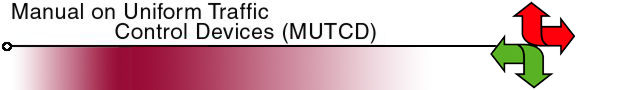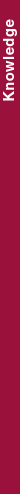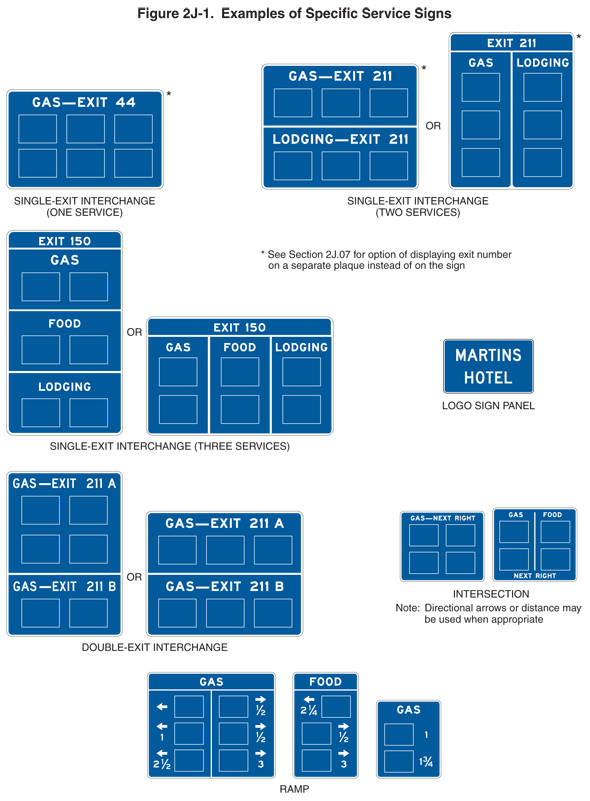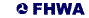Search MUTCD Site:Search 2009 Edition of MUTCD:

# 2009 Edition Part 2 Figure 2J-1. Examples of Specific Service SignsFigure 2J-1. Examples of Specific Service Signs

This figure illustrates seven examples of specific service signs. They are rectangular blue signs with white borders and lettering.

The first illustration is labeled "SINGLE-EXIT INTERCHANGE (ONE SERVICE)." It is shown as a horizontal rectangular sign with the words "GAS – EXIT 44" above six blank horizontal rectangular panels arranged in two horizontal rows of three panels each. A note states: "See Section 2J.07 for option of displaying exit number on a separate plaque instead of on the sign."

The second illustration is labeled "SINGLE-EXIT INTERCHANGE (TWO SERVICES)." It is shown as a horizontal rectangular sign divided horizontally by a white line. On the top half, the words "GAS – EXIT 211" are shown above three blank horizontal rectangular panels in one horizontal row. On the bottom half, the words "LODGING – EXIT 211" are shown above three blank horizontal rectangular panels in one horizontal row. A note states: "See Section 2J.07 for option of displaying exit number on a separate plaque instead of on the sign." To the right of this sign, the word "OR" is shown and another sign. This is shown as a vertical rectangular sign divided vertically by a white line into two sections. Above both vertical sections, a horizontal rectangular panel is shown the width of the sign with the words "EXIT 211." On the left half, the word "GAS" is shown above three blank horizontal rectangular panels in one vertical row. On the right half, the word "LODGING" is shown above three blank horizontal rectangular panels in one vertical row. A note states: "See Section 2G.07 for option of displaying exit number on a separate plaque instead of on the sign."

The third illustration is labeled "SINGLE-EXIT INTERCHANGE (THREE SERVICES)." It is shown as a vertical rectangular sign divided horizontally by three white lines into four sections. The words "EXIT 150" are shown in the top section. The remaining three sections are shown with the words "GAS," "FOOD," and "LODGING," respectively, each above two blank horizontal rectangular panels in one horizontal row. To the right of this sign, the word "OR" is shown and another sign. This is shown as a horizontal rectangular sign divided vertically by a two white lines into three sections. Above the vertical sections, a horizontal rectangular section is shown the width of the sign with the words "EXIT 150." The remaining three sections are shown with the words "GAS," "FOOD," and "LODGING," respectively, each above two blank horizontal rectangular panels in one vertical row.

The fourth illustration is labeled "LOGO PANEL" and is shown as a horizontal rectangular panel with the words "MARTINS HOTEL" on two lines.

The fifth illustration is labeled "DOUBLE-EXIT INTERCHANGE." It is shown as a vertical rectangular sign divided horizontally by a white line. On the top half, the words "GAS – EXIT 211 A" are shown above four blank horizontal rectangular panels in two horizontal rows of two panels each. On the bottom half, the words "GAS – EXIT 211 B" are shown above two blank horizontal rectangular panels arranged in one horizontal row. To the right of this sign, the word "OR" is shown and another sign. This is shown as a horizontal rectangular sign divided horizontally by a white line. On the top half, the words "GAS – EXIT 211 A" are shown above three blank horizontal rectangular panels in one horizontal row. On the bottom half, the words "GAS – EXIT 211 B" are shown above three blank horizontal rectangular panels in one horizontal row.

The sixth illustration shows two horizontal rectangular signs, both labeled "INTERSECTION."

• The sign on the left shows the words "GAS – NEXT RIGHT" above four blank horizontal rectangular panels arranged in two rows of two panels each.
• The sign on the right shows the word "GAS" above two blank horizontal rectangular panels in one vertical row to the left of the word "FOOD" above two blank horizontal rectangular panels in one vertical row. The words "NEXT RIGHT" are shown centered at the bottom of the sign under the panels. A note states: "Directional arrows and distance may be used when appropriate."

The seventh illustration shows three vertical rectangular signs labeled "RAMP":

• The first sign is shown as a vertical rectangular sign divided vertically into two sections by a white line. A horizontal rectangular panel with the word "GAS" is shown the width of the sign above both vertical sections. In the left section, three blank horizontal rectangular panels are shown in one vertical row. A short left-pointing horizontal arrow is shown to the left of each panel. The numerals "1" and "2 1/2 are shown, respectively, under the middle and bottom arrows. In the right section, three blank horizontal rectangular panels are shown in one vertical row. A short left-pointing horizontal arrow is shown to the right of each panel. The numerals "1/2," "1/2," and "3" are shown, respectively, under the top, middle, and bottom arrows.
• The second sign in the illustration is shown as a vertical rectangular sign divided vertically into two sections by a white line. The word "FOOD" is shown in the top section. Below the dividing line, three blank horizontal rectangular panels are shown in one vertical row. A short left-pointing horizontal arrow above the numeral "2 1/4" is shown to the left of the top panel. A short right-pointing horizontal arrow above the numeral "1/2" is shown to the right of the middle panel. A short right-pointing horizontal arrow above the numeral "3" is shown to the right of the bottom panel.
• The third sign in the illustration is shown as a vertical rectangular sign with the word "GAS" above two blank horizontal rectangular panels in one vertical row. The numerals "1" and "1 3/4" are shown, respectively, to the right of the top and bottom panels.

Back to Chapter 2JUnited States Department of Transportation - Federal Highway Administration